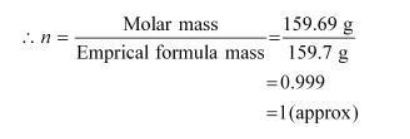# Determine the molecular formula of an oxide of iron in which the mass per cent of iron

Question.

Determine the molecular formula of an oxide of iron in which the mass per cent of iron and oxygen are $69.9$ and $30.1$ respectively. Given that the molar mass of the oxide is $159.69 \mathrm{~g} \mathrm{~mol}^{-1}$.

Solution:

Mass percent of iron (Fe) = 69.9% (Given)

Mass percent of oxygen (O) = 30.1% (Given)

Number of moles of iron present in the oxide $=\frac{69.90}{55.85}$

= 1.25

Number of moles of oxygen present in the oxide $=\frac{30.1}{16.0}$

= 1.88

Ratio of iron to oxygen in the oxide

$=1.25: 1.88$ $=\frac{1.25}{1.25}: \frac{1.88}{1.25}$

= 1 : 1.5

= 2 : 3

$\therefore$ The empirical formula of the oxide is $\mathrm{Fe}_{2} \mathrm{O}_{3} .$

Empirical formula mass of $\mathrm{Fe}_{2} \mathrm{O}_{3}=[2(55.85)+3(16.00)]$ g Molar

mass of $\mathrm{Fe}_{2} \mathrm{O}_{3}=159.69 \mathrm{~g}$Molecular formula of a compound is obtained by multiplying the empirical formula with $n$.

Thus, the empirical formula of the given oxide is $\mathrm{Fe}_{2} \mathrm{O}_{3}$ and $n$ is 1 .

Hence, the molecular formula of the oxide is $\mathrm{Fe}_{2} \mathrm{O}_{3} .$